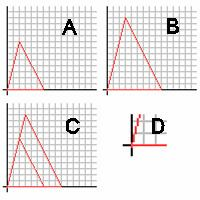# AA postulate

Updated on
Covid-19In Euclidean geometry, the AA postulate states that two triangles are similar if they have two corresponding angles congruent.

The AA postulate states that the interior angles of a triangle are always equal to 180°. By knowing two angles, such as 32° and 64° degrees, we know that the next angle is 84°, because 180-(32+64)=84. (This is sometimes referred to as the AAA Postulate—which is true in all respects, but two angles are entirely sufficient.)

The postulate can be better understood by working in reverse order. The two triangles on grids A and B are similar, by a 1.5 dilation from A to B. If they are aligned, as in grid C, it is apparent that the angle on the origin is congruent with the other (D). We also know that the pair of sides opposite the origin are parallel. We know this because the pairs of sides around them are similar, stem from the same point, and line up with each other. We can then look at the sides around the parallels as transversals, and therefore the corresponding angles are congruent. Using this reasoning we can tell that similar triangles have congruent angles.

Topics